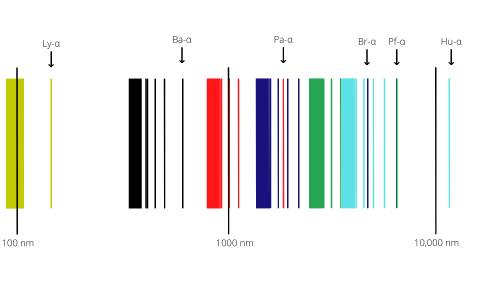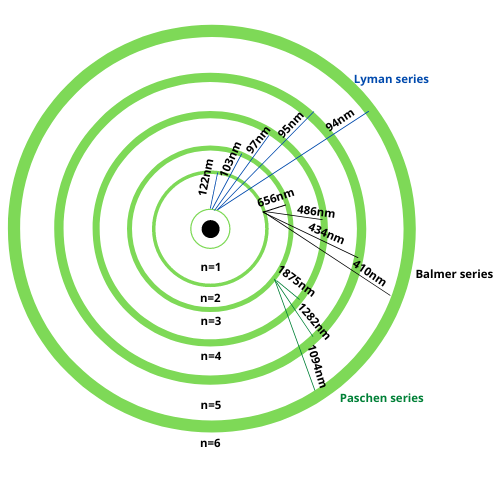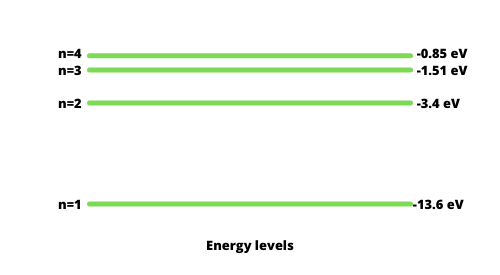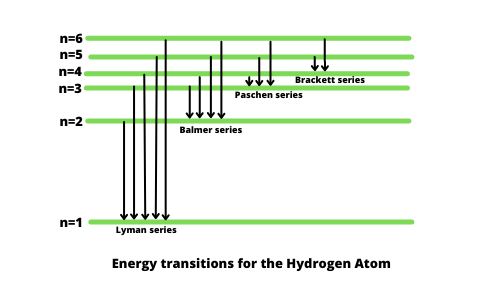GFG App
Open AppBrowser
Continue

# Spectrum of the Hydrogen Atom

Electrons in a hydrogen atom circle around a nucleus. Because of the electromagnetic force between the proton and electron, electrons go through numerous quantum states. Neil Bohr’s model helps in visualizing these quantum states as electrons orbit the nucleus in different directions.

When Electrons in an atom are supplied energy, they get excited and jump from a lower energy level to a higher energy level. As we know electrons always try to remain in their lowest energy level, the excited electrons go back to their original level and emit radiation in this process. This phenomenon explains the emission spectrum via hydrogen, sometimes known as a hydrogen emission spectrum.

We will go over an experiment to better understand what the Hydrogen emission spectrum is. Consider a thin tube carrying low-pressure gaseous hydrogen. The electrodes will then be attached to both ends of the container. Now, if we apply a high voltage to the electrode, we can see a pink glow (bright) in the tube. We know that a prism divides the light that passes through it by diffraction. The visible light spectrum is a subset of the hydrogen emission spectrum. Because the light is UV, our eyes are unable to perceive the majority of it. The electron transitioning from a high energy state to a low energy state is the primary cause of the hydrogen line emission spectrum.### What is the Spectral emission?

When an electron transitions, or jumps, from a higher energy state to a lower energy one, spectral emission occurs. To distinguish between the two states, the lower energy level is denoted by n1, while the higher energy state is denoted by n2. The energy difference between the two states corresponds to the energy difference of a photon emitted. Because the energy of each state is fixed, so is the energy difference between them, and the transition will always generate the same energy photon.Electron transitions and their resulting wavelengths for hydrogen.

The quantized energy levels of the atoms lead the spectrum to have wavelengths that represent the differences in these energy levels.### Spectral series of Hydrogen

Because the hydrogen atom is the simplest atomic system known in nature, it forms the simplest of this series. When a light or radiation beam enters the device through a slit, each individual component of the light or radiation forms an image of the source. When resolved with a spectroscope, these images can be seen. The images obtained will be in the shape of parallel lines grouped adjacent to each other with uniform spacing. When moving from the higher to lower wavelength side, the lines will be farther apart on the higher wavelength side and gradually closed on the lower wavelength side. The series limit is the shortest wavelength with the fewest separated spectral lines.

In 1885, the first such series was observed by a Swedish school teacher Johann Jakob Balmer (1825–1898) in the visible region of the hydrogen spectrum. The Balmer series is the part of the hydrogen emission spectrum that is responsible for exciting an electron from the second shell to any other shell. Other such series are mentioned below:

1. Lyman series: Electron moves to the first shell from any other shell.
2. Balmer series: Electron moves to the second shell from any other shell.
3. Paschen series: Electron moves to the third shell from any other shell.
4. Bracket series: Electron moves to the fourth shell from any other shell.
5. Pfund series: Electron moves to the fifth shell from any other shell.### Rydberg’s formula

The energy differences between levels in the Bohr model, and hence the wavelengths of emitted or absorbed photons, is given by the Rydberg formula:where,

• Z is the atomic number,
• n1 is the principal quantum number of the lower energy level,
• n2 is the principal quantum number of the upper energy level, and
• R is the Rydberg constant. (1.09677×107 m−1 for hydrogen and 1.09737×107 m−1 for heavy metals).

The wavelength will always be positive because n1 is defined as the lower level and so is less than n2. This equation is valid for all hydrogen-like species, i.e. atoms having only a single electron, and the particular case of hydrogen spectral lines is given by Z=1.

### Sample Problems

Problem 1: In a hydrogen atom, an electron undergoes a transition from the second state to the first excited state and then to the ground state. Identify the spectral series to which these transitions belong.

Solution:

Since the atom’s transition is to the first shell, it falls under the category of Layman’s series.

Problem 2: Find the ratio of wavelengths of the last line of the Balmer series and the last line of the Lyman series.

Solution:

We know that,

1/λ=Z2R[1/n12−1/n22]

For the last Balmer series

n1 = 2, n2 = ∞ , Z = 1

1/λb=R[1/22−1/∞2]

λb = 4/R

Similarly, For the last Lyman series

1/λl = R[1/12−1/∞2]

λ1 = 1/R

λb/λl = (4/R)/(1/R)

λb/λl = 4

The ratio of wavelengths of the last line of the Balmer series and the last line of the Lyman series is 4.

Problem 3: The Balmer series in the hydrogen spectrum corresponds to the transition from n1 = 2 to n2 = 3,4,… This series lies in the visible region. Calculate the wavenumber of the line associated with the transition in the Balmer series when the electron moves to n = 4 orbit.

Solution:

We know that

1/λ = Z2R(1/n12-1/n22)

Given,

n1 = 2

n2 = 4

There, the wavenumber is given as,

ν = 1/λ

ν = 109677( 1/4-1/16)

ν = 20564.44 cm-1

Problem 4: When an electron in a hydrogen atom jumps from the third excited state to the ground state, how would the de Broglie wavelength associate with the electron change?

Solution:

Given: For 3rd excited state n2 = 4,

For Ground state, n1 = 1  and Z = 1.

We know that 1/λ = Z2R(1/n12-1/n22)

1/λ = R(1/12-1/42)

1/λ = 109677 × (15/16)

λ = 16/(109677 × 15)

λ = 97 nm, which lies in the UV region

Problem 5: Calculate the shortest wavelength of light emitted in the Paschen series of the hydrogen spectrum. Which part of the electromagnetic spectrum, does it belong to? (Given : Rydberg constant, R = 107 m-1)

Solution:

In the Balmer series, an electron jumps from higher orbits to the third stationary orbit (n1 = 3).

We know that 1/λ = Z2R(1/n12-1/n22), Z=1

1/λ = R(1/32-1/n22)

For the shortest wavelength, n2 should be ∞.

1/λ = R(1/32-1/∞2)

1/λ = 107(1/9)

λ = 9 x 10-7 m

Problem 6: Calculate the shortest wavelength of photons emitted in the Bracket series of the hydrogen spectrum. Which part of the spectrum, does it belong to? [Given Rydberg constant, R = 1.1 × 107 m-1]

Solution:

In bracket series, n1 = 4,

for shortest wavelength , n2 = ∞

We know that 1/λ = Z2R(1/n12-1/n22), Z=1

1/λ = R(1/42-1/∞2)

1/λ = R/16

λ = 16/1.1 × 107

After solving we get λ = 1454 nm, which lies in the Infrared region of the spectrum.

Problem 7: The short wavelength limit for the Lyman series of the hydrogen spectrum is 913.4 A. Calculate the short wavelength limit for the Balmer series of the hydrogen spectrum.

Solution:

λl for layman series = 913.4 Å

We know that 1/λ = Z2R(1/n12-1/n22), Z=1

For the short wavelength of the Lyman series,

1/913.4 = R[1/12−1/∞2]

or

R=(1/913.4)

For a short limit of wavelength for the Balmer series,

1/λb = R[1/22−1/∞2b

=4/R

=4(913.4)

or

λb = 365.36 nm.

My Personal Notes arrow_drop_up